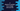# C program to check if a number is in a range with one line# Introduction :

In this C programming tutorial, we will learn how to check if a number is in a range or not using only one comparison line. The program will take all inputs from the user (number, lower range and upper range) and check if it lies in the range. We will also use one different function to test it. That will make the program more readable. Let’s take a look at the program :

## C program :

``````#include <stdio.h>
//1
#define bool int
#define true 1
#define false 0

//2
bool isInRange(int lowerLimit, int upperLimit, int no)
{
return (lowerLimit < no && no < upperLimit);
}

int main()
{
//3
int l, u, n;

//4
printf("Enter the lower limit : \n");
scanf("%d", &l);

printf("Enter the upper limit : \n");
scanf("%d", &u);

printf("Enter the number : \n");
scanf("%d", &n);

//5
if (isInRange(l, u, n) == 1)
{
printf("The number is in the range.\n");
}
else
{
printf("The number is not in the range.\n");
}

return 0;
}``````

### Explanation :

The commented numbers in the above program denote the steps number below :

1. We are defining bool as int, true as 1 and false as 0. The only reason we are doing this is for readability. Boolean is not available in C, so we can use the bool keyword to use it like boolean.
2. The function isInRange is used to find out if a number is in range or not. It takes three parameters: lower limit, an upper limit and the number itself. Note that we are using only one line inside the function to verify this. It will return true (or 1) if the number is greater than the lower limit and less than the upper limit.
3. Create three integer variables to store the user input.
4. Ask the user to enter the lower limit, upper limit and the number. Read and store them in l,u and n variable respectively.
5. Finally, check if the number is in the given range or not by using the isInRange function. It will return true which is actually 1 if the number is in range. Else, it will return false or 0.Print out the result accordingly.

### Sample output :

``````Enter the lower limit :
1
Enter the upper limit :
10
Enter the number :
4
The number is in the range.

Enter the lower limit :
1
Enter the upper limit :
10
Enter the number :
12
The number is not in the range.

Enter the lower limit :
1
Enter the upper limit :
12
Enter the number :
1
The number is not in the range.

Enter the lower limit :
1
Enter the upper limit :
12
Enter the number :
12
The number is not in the range.``````

### Conclusion :

We have learnt how to use a separate function to check if a number is in a range or not using only one line. I hope that you have found this article useful. Try to run the program and if you have any queries, drop one comment below.

You might also like :